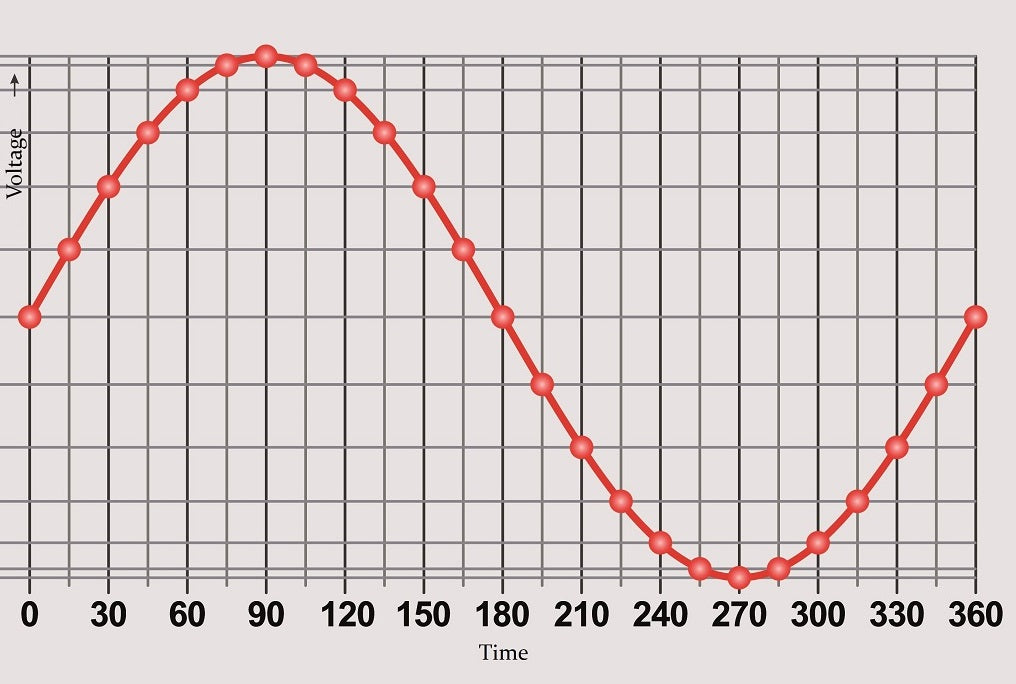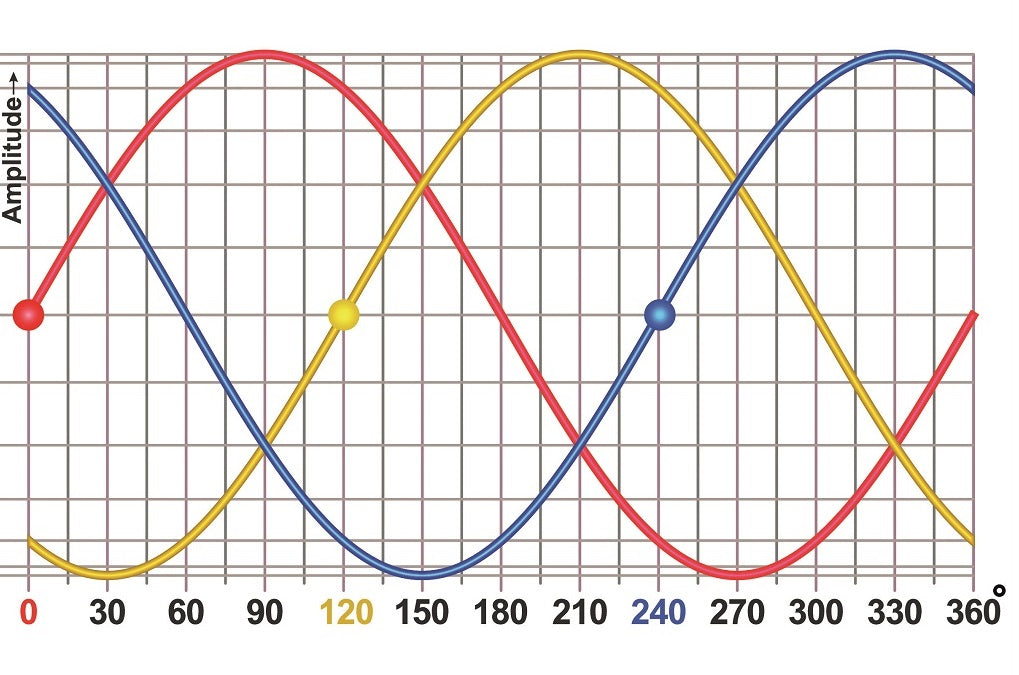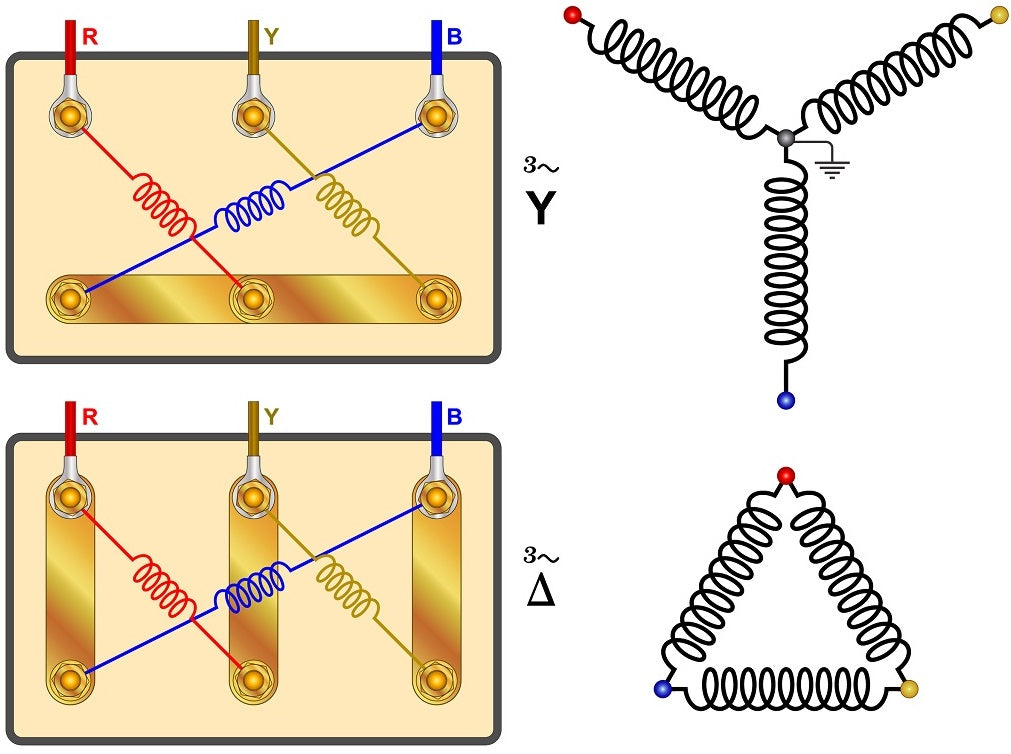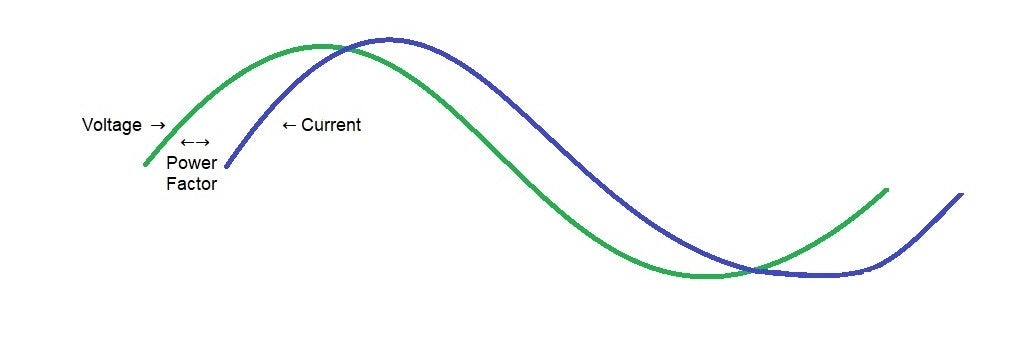# Single and Three-Phase AC Power Calculator

The measurements must be precise when choosing electrical equipment or when working with single or three-phase circuits. If you don't like to count kW and Amps manually — we have a solution! Our online AC power calculator can help you convert electrical power into current and vice-versa for single-phase and three-phase electric power.

#### Active and apparent electrical power

Apparent power, VA:

Active POWER (P), W:

#### Energy calculation

Optional. If you want to calculate the energy and electricity bill associated with this active power, fill the time value and energy cost below.

AC Energy, kWh:

Total amount of electricity bill, \$:

Below, we will teach you how to use our power calculator and tell you about formulas for these measurements. Let's dive in!

## How to Use a Power Calculator?

Do you want to convert Amps to kW (or the other way around) without doing mathematical calculations? No problem!

Our single-phase and 3-phase power calculator is elementary to use. Just fill the fields with the necessary data, including the current type, voltage, and power factor. The calculator will do everything automatically.

## AC Power Calculator — kW to Amps

• Current type

• AC - Single-Phase power calculation

• AC - Three-Phase power calculation

• DC

• Current in Amps

• Voltage type

• Line-to-line

• Line-to-neutral

• Voltage (in volts)

• Enter power factor

• Calculate; Reset; Swap;

• Power results (milliwatts)

• Power results (watts)

• Power results (kilowatts)

Ampere (A or Amp) and kilowatts (kW) are two different parameters of electricity. What do they mean?

Ampere indicates the number of currents drawn by the load. Kilowatts is the amount of consumed power by the load at any instant. In short, amperes measure the current, while kilowatts measure the power.

How can you convert amps to kilowatts for 3-phase, 1-phase alternating current (AC), or direct current (DC) electric power?

Kilowatts can't be converted to amps directly. The amount of current or power depends on the power factor, type of current, and voltage type.

However, you can get precise measurements by converting these metrics with formulas. Alternatively, you can use our kilowatts to amps three-phase converter, as well as a single-phase and DC power calculator.

## What Is Single-Phase Electric Power?Phase means the electric load distribution by either a single-phase or a three-power supply.

Single-phase electric power is typically used in domestic supplies, residential homes, and small offices. In other words, it works for appliances that need a small amount of power (refrigerators, lights, heaters, TVs, and similar tech).

The standard for single-phase power distribution in the US stands at 120 volt AC power at 60 hertz (frequency). Each hertz stands for the number of electricity changes happening within a wire each second. We should note that AC power can switch polarity, unlike DC voltage power.

### How to Calculate Single-Phase Power?

Here are the formulas you can use for single-phase power calculation.

#### Kilowatts from Amps

kW = PF × A × V / 1000

In this formula, the amount of power (in kW) is equal to the power factor of the load (PF) multiplied by the phase current measured in Amps (A), times the RMS voltage (V), and divided by 1000.

#### Amps from Kilowatts

A = 1000 × kW / (PF × V)

The A stands for phase current, which equals kW (power) multiplied by 1000, then divided by the power factor (PF) multiplied by the RMS voltage (V).

## What Is Three-Phase AC Power?Three-phase electric power is the common type of AC power generation and distribution, widely used for loads larger than 1000 watts. Unlike single-phase power supply, 3-phase power needs less aluminum or copper, has a greater conductor efficiency, and sustains large power loads. It also provides an overall greater density, thus optimizing energy consumption.

For more accurate power calculation, a formula for 3-phase applications must account the type of power configuration. The two most common configurations are delta (used only three wires) and wey (has a fourth neutral wire).

A three-phase electric power supply is typically found in commercial and industrial facilities with large motors, manufacturing equipment, capacity air conditioners, and other heavy-load applications.

Now, for the main topic. How do you transfer amps to kilowatts in three-phase circuit (and vice-versa)?### 3 Phase Power Formula Calculation

Here are the equations you can use for three-phase power calculation. Keep in mind that the 3-phase power formula will be different for line-to-line and neutral voltages.

#### Kilowatts from Amps (line-to-line voltage)

kW = √3 × PF × A × V / 1000

The power (kW) equals the square root of three (√3) multiplied by the power factor (PF) times the current (Amps or A), times the line-to-line RMS voltage (V) divided by 1000.

#### Kilowatts from Amps (line-to-neutral voltage)

kW = 3 × PF × A × V / 1000

You can calculate 3-phase power from amps to kW with line-to-neutral voltage just as you would with line-to-line voltage. The only difference is that the square root of three (√3) is replaced by a number three (3), and line-to-line RMS is replaced by line-to-neutral RMS voltage in the equation.

#### Amps from Kilowatts (line-to-line voltage)

A = 1000 × kW / (√3 × PF × V)

The phase current (A) equals 1000 times the kilowatts (kW), divided by the square root of three multiplied by the power factor (PF), multiplied by the line-to-line RMS voltage (V).

#### Amps from Kilowatts (line-to-neutral voltage)

A = 1000 × kW / (3 × PF × V)

For a 3-phase power supply calculation, you need to multiply 1000 by the power (kW), divided by three times the power factor times line-to-neutral RMS voltage (V).

## What Is the Power Factor?Now, we've mentioned the power factor (PF) several times in the formulas. It refers to the ratio between the real and apparent power dissipated by an AC circuit to the electrically powered product.

Real power means electrical power used by the devices, while apparent power (reactive power) means electricity supplied to the AC circuit.

The power factor value ranges from zero to one, depending on the resistive and real load.

• The power factor stands at zero (0) when all power is reactive power.

• The power factor stands at one (1) when all power is real (without reactive power).

### How to Calculate Power Factor?

There are many PF equations based on the type of power and current. Let's go through every power factor formula.

The power factor for sinusoidal current is equal to the absolute cosine value of the apparent power phase. The apparent power phase angle will be marked as φ in the formulas below.

#### To calculate real power in watts:

W = |VA| × PF = |VA| × |cos φ|

The real power equals apparent power in volt-ampere (VA) multiplied by the PF factor.

PF(resistive load) = P / |S| = 1

The real power in resistive impedance loads equals apparent power (S), with the power factor (PF) standing at 1 (one).

#### Volt-amps reactive POWER

Q = |VA| × |sin φ|

The reactive power (Q) in volt-amperage reactive equals the apparent power in volt-ampere (VA) multiplied by the sine of the phase angle.

#### Single-phase power FORMULA

PF = |cos φ| = 1000 × kW / (V × A)

To calculate the power factor from a single-phase circuit, you need to multiply 1000 by the power in kilowatts (kW), divided by RMS voltage (V) times phase current in amperes (A).

#### 3-phase power FORMULA (line-to-line)

PF = |cos φ| = 1000 × kW / (√3 × V × A)

Line-to-line 3-phase power calculation for power factor is 1000 multiplied by power in kilowatts (kW), then divided by the square root of three multiplied by the line-to-line RMS voltage (V) times phase current in amps (A).

#### 3-phase power (line-to-neutral)

PF = |cos φ| = 1000 × kW / (3 × V × A)

To measure the PF for line-to-neutral 3-phase power, multiply 1000 by kilowatts (kW), then divide by three times line-to-neutral RMS voltage (V) times amps (A).

## Converting kW to Amps

Do you want to convert kilowatts to amps? It's possible to calculate this data using a simple formula (provide you know the power factor). The formula is:

​I = P / (√3 × PF × V)​

In this equation, I stands for the current (amps), P stands for the related power (measured in watts), PF is the power factor, and V is the voltage.

If your power measures in thousands of watts, it'll be easier to convert data to watts by multiplying it by 1000. You also need to make sure your voltage measures in kilovolts (kV).

Let's give you an example using the formula above. If your power factor is 0.8, and you have 1.5 kW (1500 W) power and a steady 220 voltage (V), the calculation will be:

I = 1,500 / (√3 × 0.8 × 220) = 4.92 A

Just like that, you can convert watts and kilowatts to amperes.

## Converting Amps to kW

Now, let's do the reverse. For amps to kilowatt conversion, use the following formula:

​P = √3 × PF × I × V

The markings are the same here. P is power, the power factor is PF, I is the current (amps), and V stands for voltage.

For our next example, we will use the same voltage (220 V) and power factor (0.8) and have a 4.92 A current. Now, let's convert amps to kilowatts:

P = √3 × 0.8 × 4.92 × 220 = 1500 W = 1.5 kW

## Conclusion

As you can see, calculating and converting amps to kilowatts and vice-versa is pretty easy. However, it can be a little time-consuming to use formulas for 3-phase apparent power calculation.

If you want to get precise measurements without any hassle — use our online AC power calculator, as it will help you find the best electrical supplies for your systems.

Blackhawk Supply has a wide assortment of HVAC, plumbing, and electrical equipment. Choose relaysenclosurestransformerspower supplies, and other devices!

Free Shipping On
Orders over \$999*

Excellent Customer
Satisfaction

Secure Online
Payment

5-star Customer
Service

Quantity
Discounts